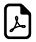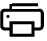Two Times Tables - Double Your Pleasure2 Times Multiplication Table Trick

An online two times table tricks.Easy way to learn the 2 times table.Two Times Table Easy Learningcan write to
can write to
can write to
can write to
can write to
can write to
can write to
can write to
can write to
can write to
can write to

How 2 times table works?

When you multiply a number by 2, you just double that number. Thats some pretty simple math, even for the most numerically challenged of us. So, if you want to figure out what 3 x 2 is, you just add 3 + 3 (the answer is 6, by the way). Any number times two is the same as that number PLUS itself. Here one more example: 10 x 2 is the same as 10 + 10, which equals 20. Practice a couple - its really easy.

Think 2 times.Think Double !!!

What math times time do you want to generate ?Times Table Tricks 2 to 11►

2 Times Table Trick

4 Times Table Trick

5 Times Table Trick

6 & 7 Times Table Trick

8 Times Table TrickTop Calculators ►

Tax Calculator, Calories Burned Calculator, PERT, SD Calculator, Dog Age Calculator, Children Equal Playing Time Calculator